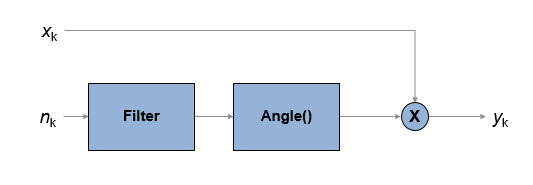# Phase Noise

Apply receiver phase noise to complex baseband signal

•Libraries:
Communications Toolbox / RF Impairments and Components

## Description

The Phase Noise block adds phase noise to a complex signal. This block emulates impairments introduced by the local oscillator of a wireless communication transmitter or receiver. The block generates filtered phase noise according to the specified spectral mask and adds it to the input signal. For a description of the phase noise modeling, see Algorithms.

## Ports

### Input

expand all

Input signal, specified as an NS-by-1 numeric vector or NS-by-M numeric matrix. NS represents the number of samples and M is the number of channels.

Data Types: `double` | `single`
Complex Number Support: Yes

### Output

expand all

Output signal, returned as a complex-valued signal with the same data type and size as the input signal.

## Parameters

expand all

Phase noise level in decibels relative to carrier per hertz (dBc/Hz), specified as a vector of negative scalars. The Phase noise level (dBc/Hz) and Frequency offset (Hz) parameters must have the same length.

Frequency offset in Hz, specified as a vector of positive increasing values. The maximum frequency offset value must be less than FS / 2, where FS represents the Sample rate (Hz) parameter value.

The Phase noise level (dBc/Hz) and Frequency offset (Hz) parameters must have the same length.

Sample rate in Hz, specified as a positive scalar greater than two times the maximum value specified by the Frequency offset (Hz) parameter.

Initial seed of noise generator, specified as a positive scalar.

This block uses the Random Source block to generate noise. The block generates random numbers using the Ziggurat method (V5 RANDN algorithm). Every time you rerun the simulation, the block reuses the same initial seed. That way, the block outputs the same signal each time you run a simulation.

Display magnitude response of filter defined by the Phase Noise block. The block uses the FVTool function to display the magnitude response.

Type of simulation to run, specified as ```Interpreted execution``` or `Code generation`.

• `Interpreted execution` — Simulate the model by using the MATLAB® interpreter. This option requires less startup time, but the speed of subsequent simulations is slower than with the ```Code generation``` option. In this mode, you can debug the source code of the block.

• `Code generation` — Simulate the model by using generated C code. The first time you run a simulation, Simulink generates C code for the block. The model reuses the C code for subsequent simulations unless the model changes. This option requires additional startup time, but the speed of the subsequent simulations is faster than with the `Interpreted execution` option.

## Block Characteristics

 Data Types `double` | `single` Multidimensional Signals `no` Variable-Size Signals `no`

## Algorithms

expand all

The output signal, yk, is related to input sequence xk by yk=xkejφk, where φk is the phase noise. The phase noise is filtered Gaussian noise such that φk=f(nk), where nk is the noise sequence and f represents a filtering operation.To model the phase noise, define the power spectrum density (PSD) mask characteristic by specifying scalar or vector values for the frequency offset and phase noise level.

• For a scalar frequency offset and phase noise level specification, an IIR digital filter computes the spectrum mask. The spectrum mask has a 1 / f characteristic that passes through the specified point. For more information, see IIR Digital Filter.

• For a vector frequency offset and phase noise level specification, an FIR filter computes the spectrum mask. The spectrum mask is interpolated across log10(f). For more information, see FIR Filter.

 Kasdin, N. J., "Discrete Simulation of Colored Noise and Stochastic Processes and 1/(f^alpha); Power Law Noise Generation." The Proceedings of the IEEE. Vol. 83, No. 5, May, 1995, pp 802–827.